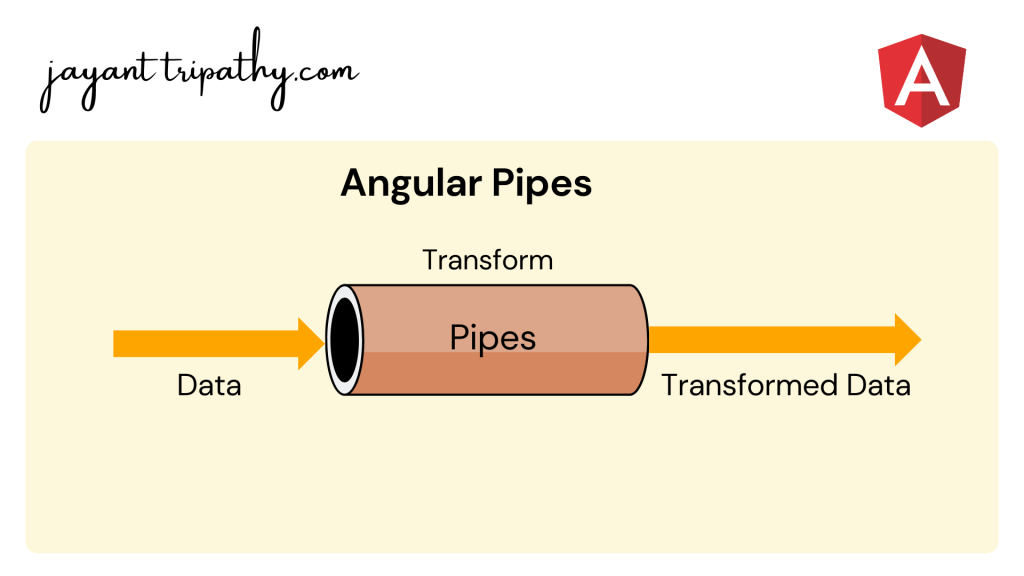# Pipes in AngularIn this article we are going to discuss about Pipes in Angular. Here we will discuss what a pipe is, why it is important in Angular, and how many different types of pipes we can use in Angular. Please read my previous article ngClass Directive in Angular.

## What are Pipes in Angular?

Angular Pipes takes data as input and formats or transform the data to display in the template. We use them to change the appearance of the data before presenting it to the user. The most common application of pipes is to display dates in the correct format for the user’s locale.

Using the Pipe `(|)` operator, we can apply the pipes features to any of the property in angular application. There are so many built-in pipes provides by Angular Framework such as lowercase, uppercase, titlecase, decimal, date, percent, currency etc. It is also possible to create custom pipes etc.

## How many types of Pipes in Angular?

We can use Pipes in two ways.

• Built-in-Pipe : There are some built-in pipes provides by Angular Framework such as lowercase, uppercase, titlecase, decimal, date, percent, currency etc..
• Parametrized
• Chaining
• Custom Pipe

## Angular Pipes Syntax

The syntax of the pipe is as follows.

• `Expression`: is the expression, which you want to transform
• `|` : is the Pipe Character
• `pipeOperator` : name of the Pipe
• `pipeArguments`: arguments to the Pipe
``Expression | pipeOperator[:pipeArguments]``

On the below image we used the built-in Pipe to make uppercase letter. Here we use Angular Interpolation to pass the input data as “jayant” and with the `pipe(|)` symbol use the pipe as uppercase then it will give result in uppercase “JAYANT”.

## Angular Pipe Examples

Let’s see some basic angular pipe examples,

Date Format with Pipe

• We declared the current date in the typescript file.
• On the HTML markup use the Angular Interpolation to render the date.
• We use one without the angular pipe and the other with it. You can see how it formatted the date as a result.
``````export class AppComponent {
currentDate: Date = new Date();
}
``````
``````<p>Current Date Without Formatting : {{ currentDate }} </p>

<p>Current Date Using Pipe Formatting : {{ currentDate | date }} </p>``````

Date Format with Pipe using Expression

Synatx:

• `date_expression` is a date object or a number
• `date` is the name of the pipe
• `format` is the date and time format string which indicates the format in which date/time components are displayed.
``date_expression | date[:format]``
``````  <p>LongDate : {{currentDate | date:'longDate'}} </p>
<p>medium : {{currentDate | date:'medium'}} </p>
<p>mediumDate : {{currentDate | date:'mediumDate'}} </p>
<p>mediumTime : {{currentDate | date:'mediumTime'}} </p>
<p>short : {{currentDate | date:'short'}} </p>
<p>shortDate : {{currentDate | date:'shortDate'}} </p>
<p>fullDate : {{currentDate | date:'fullDate'}} </p>
<p>dd-MM-y : {{currentDate | date:'dd-MM-y'}} </p>
<p>dd-MM-yy HH:mm : {{currentDate | date:'dd-MM-yy HH:mm'}} </p>``````

DecimalPipe / NumberPipe

The Decimal Pipe is used to Format a number as Text. This pipe will format the number according to locale rules.

Syntax

• number_expression is the number you want to format
• number is the name of the pipe
• digitInfo is a string which has the following format
``number_expression | number[:digitInfo]``
``````export class AppComponent {

num: number= 5656.442566;

}``````
``````  <p> Unformatted Number : {{num}}</p>
<p> Formatted Number : {{num | number}}</p>
<p> Formatted Number : {{num | number:'3.1-2'}}</p>
<p> Formatted Number : {{num | number:'7.1-5'}} </p>``````

CurrencyPipe

Formats a number as currency using locale rules.

Syntax

``number_expression | currency[:currencyCode[:symbolDisplay[:digitInfo]]]``
• number_expression currency to format a number as currency.
• Currency is the name of the pipe
• currencyCode is the ISO 4217 currency code, such as USD for the US dollar and EUR for the euro.
• symbolDisplay is a Boolean indicating whether to use the currency symbol or code. Use true to display symbol and false to use code
• digitInfo is similar to the one used in decimal pipe
``````export class AppComponent {

value: number= 1021;

}``````
``````  <p>Unformatted : {{value}} </p>
<p>Currency converted Dollar : {{value | currency }} </p>
<p>Currency converted Rupees : {{value | currency:'INR':true:'4.2-2'}} </p>
<p>Currency converted Euro : {{value | currency:'EUR':true:'4.2-2'}} </p>``````

PercentPipe

Formats the given number as a percentage according to locale rules.

• number_expression is the number you want to format
• percent is the name of the pipe
• digitInfo is a string which has the following format. It is similar to used in decimal pipe
``number_expression | percent[:digitInfo]``
``````export class AppComponent {
per: number= 74.14;
}``````
``````  <p>Unformatted :{{per}} </p>
<p>Example 1 :{{per | percent }} </p>
<p>Example 2 :{{per | percent:'2.1-2'}} </p>``````

SlicePipe

Creates a new List or String containing a subset (slice) of the string or array.

Syntax

The slice pipes take two arguments. The first argument start is the starting index of the string/array. The second argument end is the ending index of the string/array. If the start or end index is negative then the index is counted from end of the string/array.

• array_or_string_expression is the string to slice.
• slice is the name of the pipe.
• start is the start position/index from where the slicing will start.
• end is the ending index/position in the array/string.
``array_or_string_expression | slice:start[:end]``

``````export class AppComponent {

myText: string= 'Hi Welcome to jayant.tripathy.com';

}
``````
``````  <p>Complete String : <span style="color: red;"> {{myText}} </span> </p>

<p>Complete String Length : {{myText.length}} </p>

<p>Example 1 : <span style="color: purple;"> {{myText | slice:13:20}} </span></p>

<p>Example 2 : <span style="color: blue;"> {{myText | slice:-19}} </span></p>``````

Conclusion

In this article we discussed about Pipes in Angular. We discussed what a pipe is, why it is important in Angular, and how many different types of pipes we can use in Angular.